# Automatic Generation of Auxiliary Functions

Automatically generated auxiliary function sets are provided in deMon2k by means of the auxiliary function specification GEN-A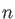, with, and GEN-A*, with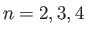[133,156]. The GEN-A1 set possesses onlyauxiliary functions and is usually used only for debugging. The GEN-A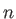sets consist of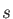,, and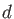Hermite Gaussian functions. In addition, the GEN-A* also have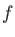andHermite Gaussians. Because the auxiliary functions are used to fit the electron density they are grouped in,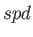, and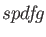sets. The exponents are shared within each of these sets [53,54]. Therefore, the auxiliary function notation (3,2,2) describes 3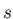sets with a total of 3 functions, 2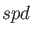sets with a total of 20 functions and 2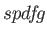sets with a total of 70 functions (see also 4.3.3). The range of exponents of all automatically generated auxiliary functions is determined by the smallest,, and largest,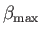, primitive Gaussian exponent of the specified orbital basis set. Therefore, the GEN-Aand GEN-A* auxiliary function sets differ for different orbital basis sets. The number of exponents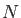(auxiliary function sets) is given by: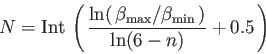(1)

Hereis 1, 2, 3, or 4 according to the index of the selected GEN-Aor GEN-A* set. The exponents are generated in almost even-tempered form (see details below) and split into,and, if a GEN-A* set is specified,sets. The tightest (largest) exponents are assigned to thesets, followed by the, and, if specified,sets. The basic exponent from which the generation starts is defined as:(2)

In case ECPs or MCPs are used, equation (A..2) changes to:(3)

Furthermore, onlyandsets are generated on ECP and MCP centers. Fromthe two tightest, orset exponents,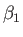andare generated according to the formulas: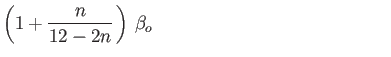(4)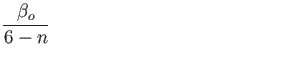(5)

The otherorset exponents are generated according to the even-tempered progression: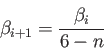(6)

The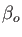exponent of the subsequentsets is also generated according to the progression (A..6). Based on thisexponent, the exponents of the first twosets are calculated with the formulas (A..4) and (A..5). The subsequentset exponents are calculated again according to the even-tempered progression (A..6). In the same way, theset exponents are calculated. In the case of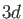elements, an extra diffuseauxiliary function set is added.